Download the eSaral app and start learning from Kota's top IITians and doctors.

# NCERT Solutions for Class 9 Maths chapter 13 Exercise 13.4 - Surface Areas and Volumes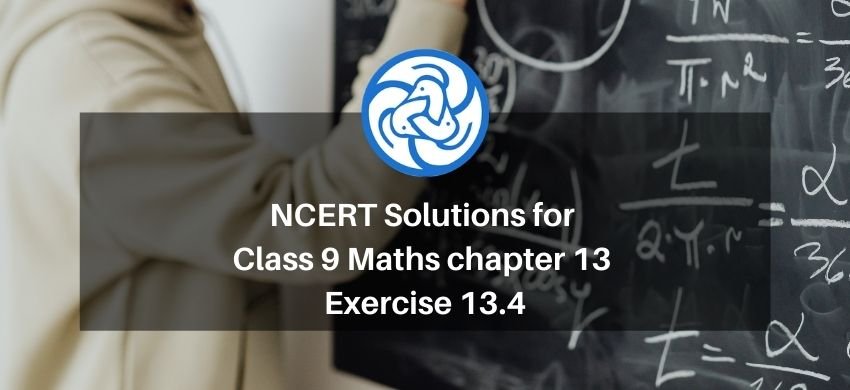Hey, are you a class 9 Student and Looking for Ways to Download NCERT Solutions for Class 9 Maths chapter 13 Exercise 13.4? If Yes then you are at the right place.

Here we have listed Class 9 maths chapter 13 exercise 13.4 solutions in PDF that is prepared by Kota’s top IITian’s Faculties by keeping Simplicity in mind.

If you want to score high in your class 9 Maths Exam then it is very important for you to have a good knowledge of all the important topics, so to learn and practice those topics you can use eSaral NCERT Solutions.

So, without wasting more time Let’s start.

### Download The PDF of NCERT Solutions for Class 9 Maths chapter 13 Exercise 13.4 "Surface Areas and Volumes"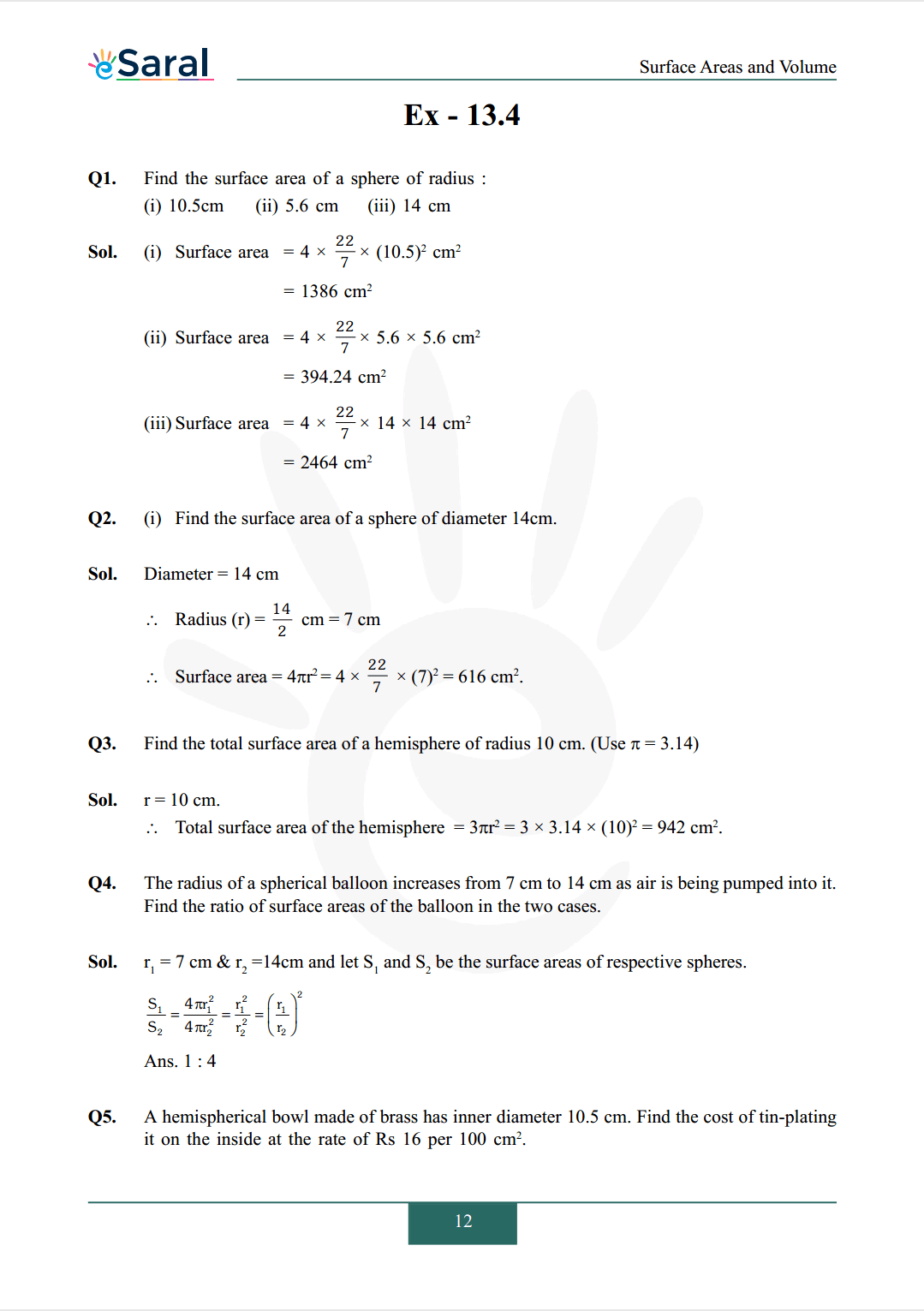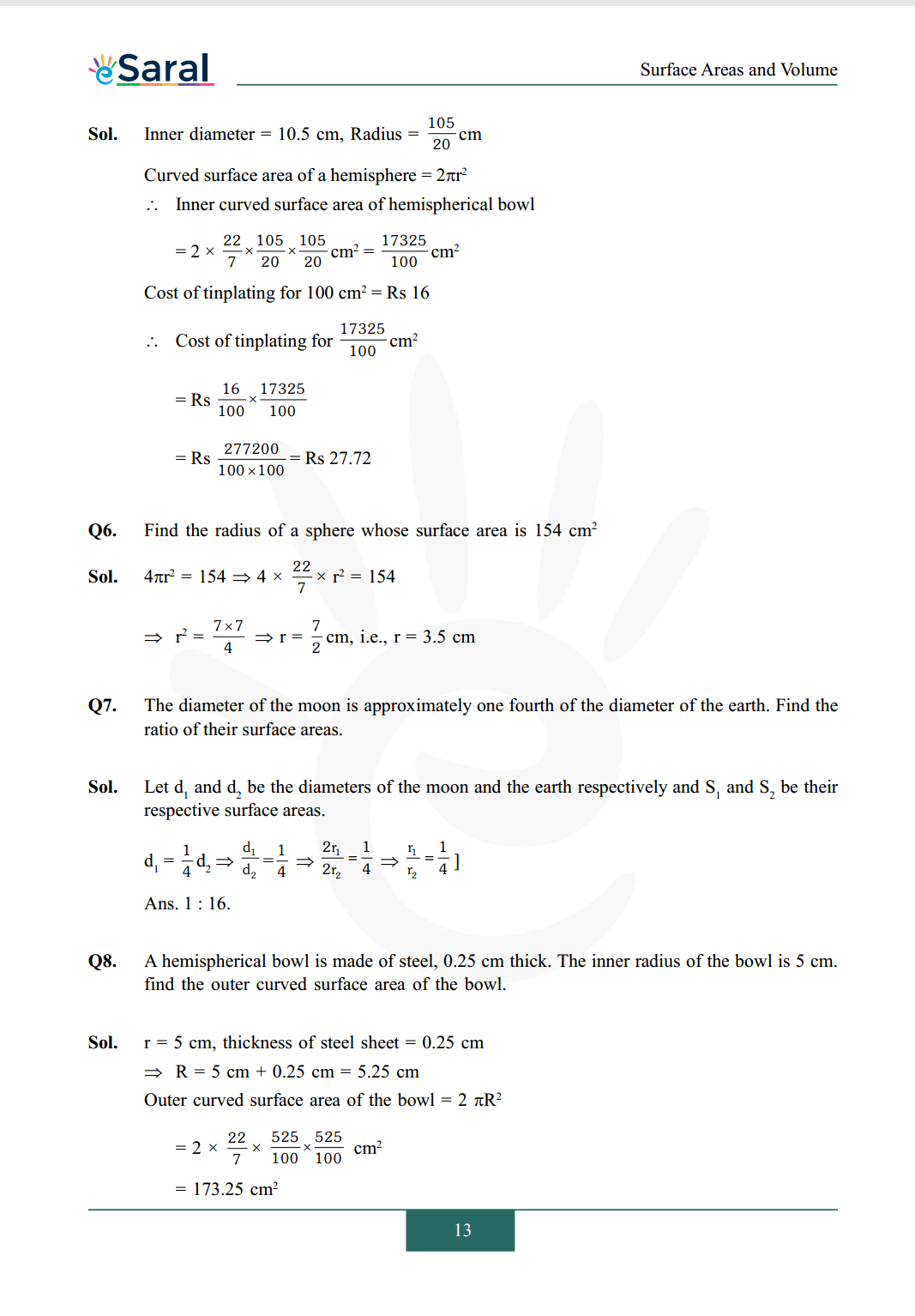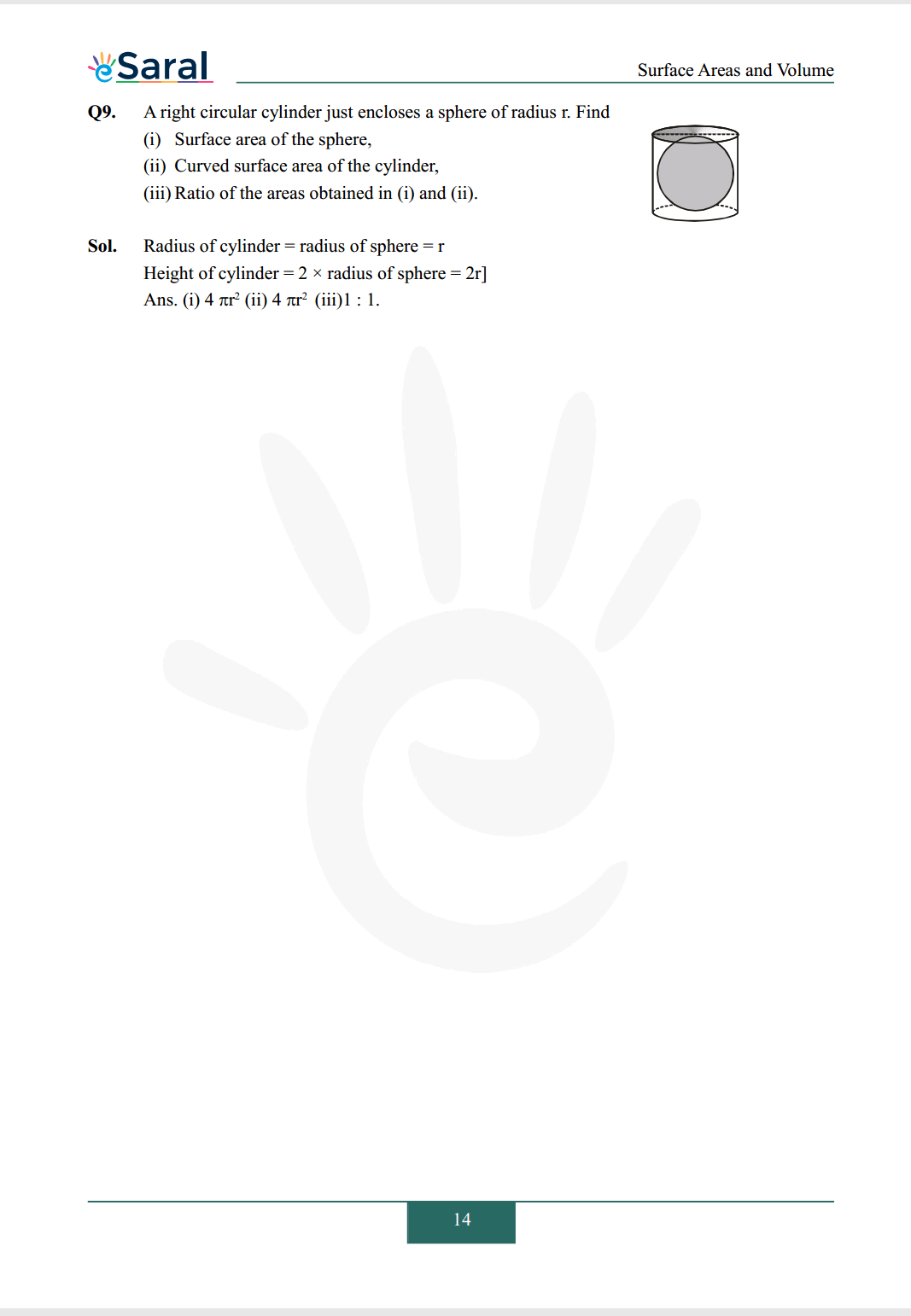#### All Questions of Chapter 13 Exercise 13.4

Once you complete the chapter 13 then you can revise Ex. 13.4 by solving following questions

Q1. Find the surface area of a sphere of radius:
(i) $10.5 \mathrm{~cm}$
(ii) $5.6 \mathrm{~cm}$
(iii) $14 \mathrm{~cm}$

Q2. (i) Find the surface area of a sphere of diameter 14cm.

Q3. Find the total surface area of a hemisphere of radius $10 \mathrm{~cm}$. (Use $\pi=3.14$)

Q4. The radius of a spherical balloon increases from 7 cm to 14 cm as air is being pumped into it. Find the ratio of surface areas of the balloon in the two cases.

Q5. A hemispherical bowl made of brass has inner diameter $10.5 \mathrm{~cm}$. Find the cost of tin-plating it on the inside at the rate of $\mathrm{Rs} 16$ per $100 \mathrm{~cm}^{2}$.

Q6. Find the radius of a sphere whose surface area is $154 \mathrm{~cm} ^ {2}$

Q7. The diameter of the moon is approximately one fourth of the diameter of the earth. Find the ratio of their surface areas.

Q8. A hemispherical bowl is made of steel, $0.25 \mathrm{~cm}$ thick. The inner radius of the bowl is $5 \mathrm{~cm}$. find the outer curved surface area of the bowl.

Q9. A right circular cylinder just encloses a sphere of radius $\mathrm{r}$. Find
(i) Surface area of the sphere,
(ii) Curved surface area of the cylinder,
(iii) Ratio of the areas obtained in (i) and (ii).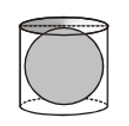NCERT Class 9 Maths Book Free PDF

NCERT Class 9 Maths Exemplar Free PDF

Complete Solutions for Class 9 Maths chapter 13 Free PDF

Class 9 Maths Chapter 12 Exercise 12.1 Free Solutions PDF

Class 9 Maths Chapter 12 Exercise 12.2 Free Solutions PDF

If you have any Confusion related to NCERT Solutions for Class 9 Maths chapter 13 Exercise 13.4 then feel free to ask in the comments section down below.

To watch Free Learning Videos on Class 9 by Kota’s top Faculties Install the eSaral App